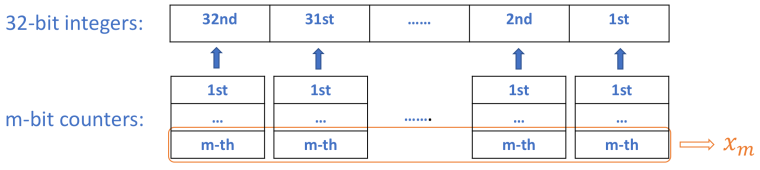### Single Number

Given a non-empty array of integers, every element appears twice except for one. Find that single one.

Note:

Your algorithm should have a linear runtime complexity. Could you implement it without using extra memory?

Example 1:

Input: [2,2,1]
Output: 1
Example 2:

Input: [4,1,2,1,2]
Output: 4

### single number II

Given a non-empty array of integers, every element appears three times except for one, which appears exactly once. Find that single one.

Note:

Your algorithm should have a linear runtime complexity. Could you implement it without using extra memory?

Example 1:

Input: [2,2,3,2]
Output: 3
Example 2:

Input: [0,1,0,1,0,1,99]
Output: 99### single number III

Given an array of numbers nums, in which exactly two elements appear only once and all the other elements appear exactly twice. Find the two elements that appear only once.

Example:

Input: [1,2,1,3,2,5]
Output: [3,5]

Note:

The order of the result is not important. So in the above example, [5, 3] is also correct.
Your algorithm should run in linear runtime complexity. Could you implement it using only constant space complexity?

python实现的代码如下:

### 总结

1. 用求和的方式是通用的解法
2. 位操作方法是有局限的，对于不同的变种有不同解法，用位操作解决的关键是
3. 利用相同的数偶数次按位亦或结果为0，计数次按位亦或是自身